# Addition and Subtraction Word Problem Keywords

Addition and Subtraction Word Problem Keywords• Each word problem contains numbers which should be written down.
• Keywords in the word problem can help us to decide whether to add or subtract these numbers.
• The list above contains some common addition and subtraction keywords.
• If you see these words in the word problem, they may help you to decide whether to add or subtract the numbers that you have already written down.

Write out the numbers given in the word problem text.

Decide whether to add or subtract these numbers depending on the keywords in the question.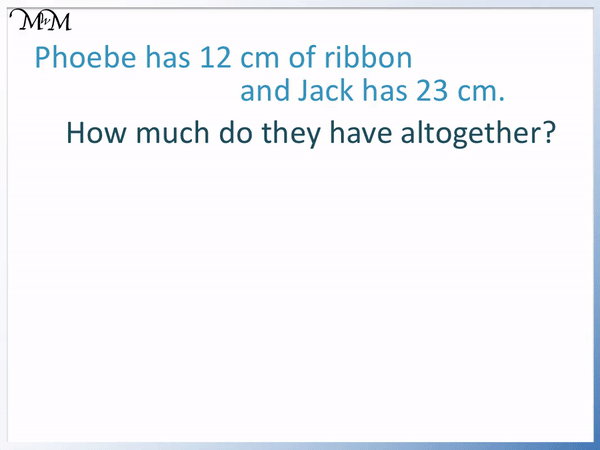• Phoebe has 12 cm of ribbon and Jack has 23 cm of ribbon.
• How much ribbon do they have altogether?
• The word ‘altogether’ tells us to add the two numbers to make a total.
• We can write the numbers of 12 and 23 with their digits above each other.
• Adding the units column, 2 + 3 = 5.
• Adding the tens column, 1 + 2 = 3.
• 12 + 23 = 35 and so, there is 35 cm of ribbon in total.# Mixed Addition and Subtraction Word Problems

Addition and subtraction word problems are commonly taught in Year 2 (Key Stage 1 in the UK) or second grade (in the USA).

The strategy to solve word problems is to firstly, write out the numbers involved and secondly, to decide which operation to use by reading the keywords in the question.

To solve addition and subtraction word problems, we try to read the question and look for keywords. The keyword list below will help to identify whether we have an addition or subtraction word problem.• Plus
• More
• Total
• Increase
• Together / Altogether
• Combined
• Sum
• Grow

If we see these words, we likely have an addition word problem.

Some common subtraction keywords are:

• Subtract
• Minus
• Take away
• Less / Fewer than
• Difference
• Decrease
• How many are left / remain?
• Change – in money questions
• Words ending in ‘er’, such as shorter, longer, faster.

Here is our first example of a word problem.

William has 20 counters and is given 7 more.

How many does he have in total?

We can see that we have the two addition keywords which are: ‘more‘ and ‘total‘.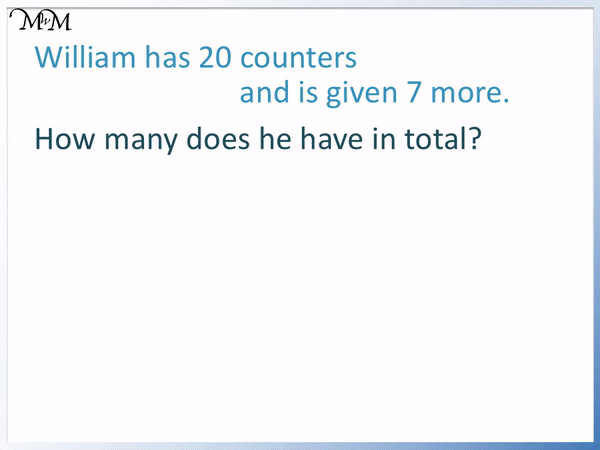When teaching word problems, it is useful to first write out the numbers that are in the text of the question.

We have 20 and 7.

The words ‘more‘ and ‘total‘ tell us that this is an addition word problem. We start with 20 counters and add 7 more.

Once we know that we have an addition word problem, then we can add the numbers.

20 + 7 = 27William has 27 counters in total.

Here is another word problem example.

Phoebe has 12 cm of ribbon and Jack has 23 cm.

How much do they have altogether?

Our strategy is to first write out the numbers involved in the question.

We write down 12 and then 23. We can write the numbers above each other and line up the digits in each number.There is only one keyword in this question which is altogether.

This in an addition keyword which tells us that we want to combine the two amounts to make a total.

We want to add the numbers 12 and 23.

It is common for children to write down the units involved in the question at this stage. However, it is easiest to just write down the numbers themselves and then to put the units in at the end of the question as part of checking the working out.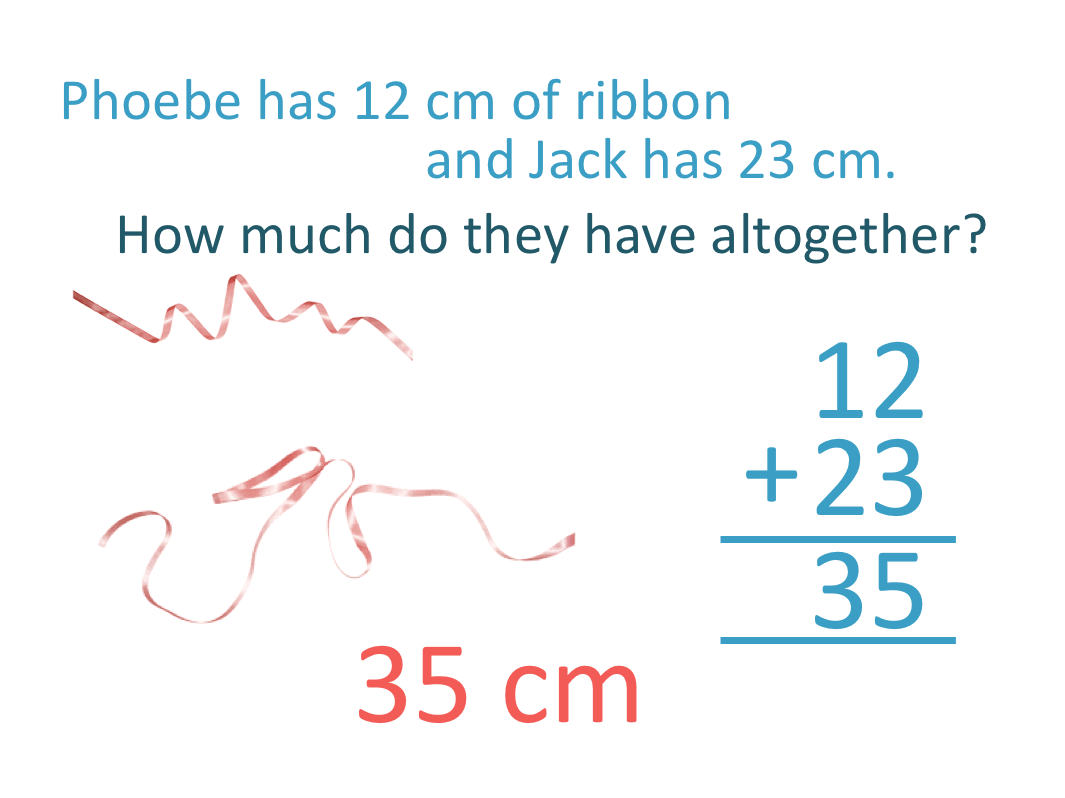Adding the units column, 2 + 3 = 5.

Adding the digits in the tens column, 1 + 2 = 3.

Therefore 12 + 23 = 35 and so, we have 35 cm of ribbon in total.

We are measuring the length of ribbon in cm and so, we write ‘cm’ at the end of our answer.

Here is another word problem example.

I buy 2 sweets that cost 43 pence each.

How much do they cost in total?

Each sweet costs 43 pence and there are two of them.

We write down 43 twice in this question.The keyword ‘total‘ tells us that this is an addition word problem.

We will add the two 43 amounts by writing their digits directly above each other without writing ‘pence’ at the end.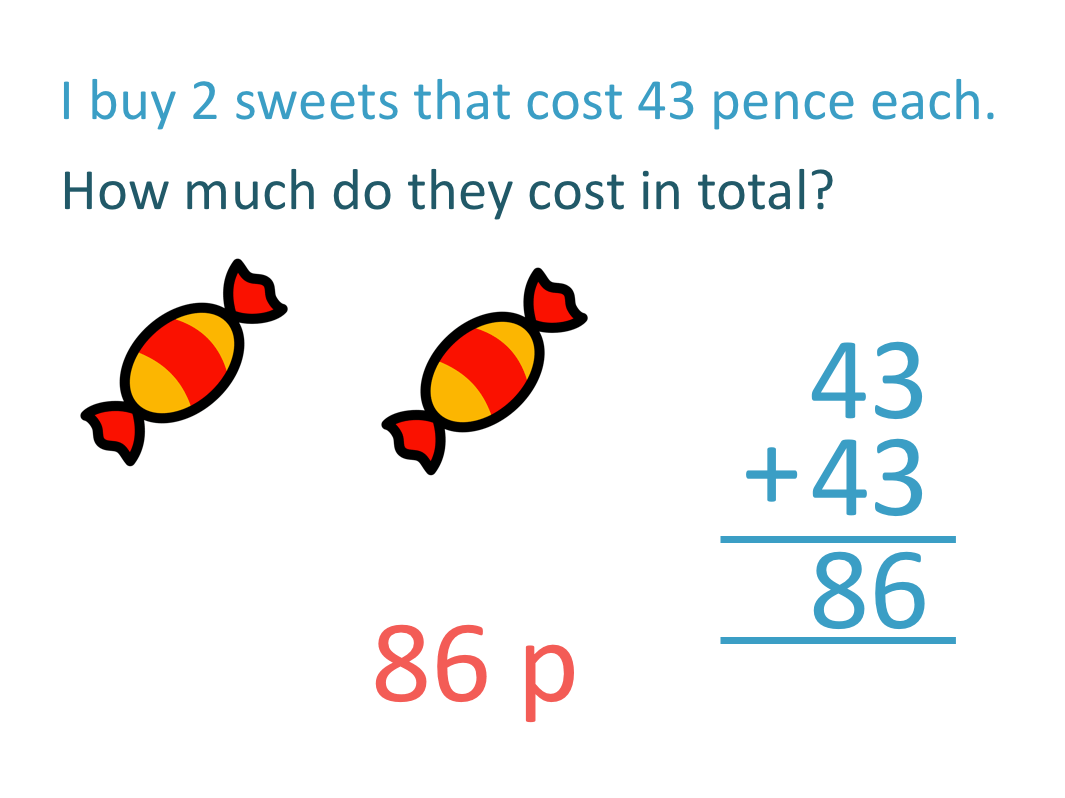Adding the units, 3 + 3 = 6.

Adding the tens, 4 + 4 = 8.

The two sweets cost 86 pence in total.

We can write the pence or ‘p’ on the final answer now that the calculation has been done.

In this worded question, we have only one number in the text itself. There is only one ’43’ written.

It can help to draw a diagram when teaching word problems to children to help picture the situation.

Here is another word problem involving money.

Matthew has 35 pence.

He spends 13 pence.

How much does he have left?

We write down the numbers involved, which are 35 and 13.

In this word problem, the keyword is left.

Finding how much is left is a keyword for a subtraction word problem.

This means that we subtract the smaller number from the larger number.To subtract 13 from 35, we write the larger number above the smaller number and line up the digits.

Subtracting the units column, 5 – 3 = 2.

Subtracting the tens column, 3 – 1 = 2.

35 – 13 = 22This was a problem involving finding change with money.

Spending money and then receiving change is also very likely to indicate that a word problem is a subtraction one.

In this next worded problem, Adam has 59 grams of chocolate.

He eats 49 grams.

How much does he have left?

The first step of the word problem strategy is to write out both of the numbers involved in the question.

We have 59 and 49.

The second step is to identify keywords. The word left is a subtraction keyword.

We want to see how much is left after 49 grams have been subtracted.We write the subtraction with the larger number above the smaller number.

Subtracting the units column digits, 9 – 9 = 0.

Subtracting the tens column digits, 5 – 4 = 1.

59 – 49 = 10.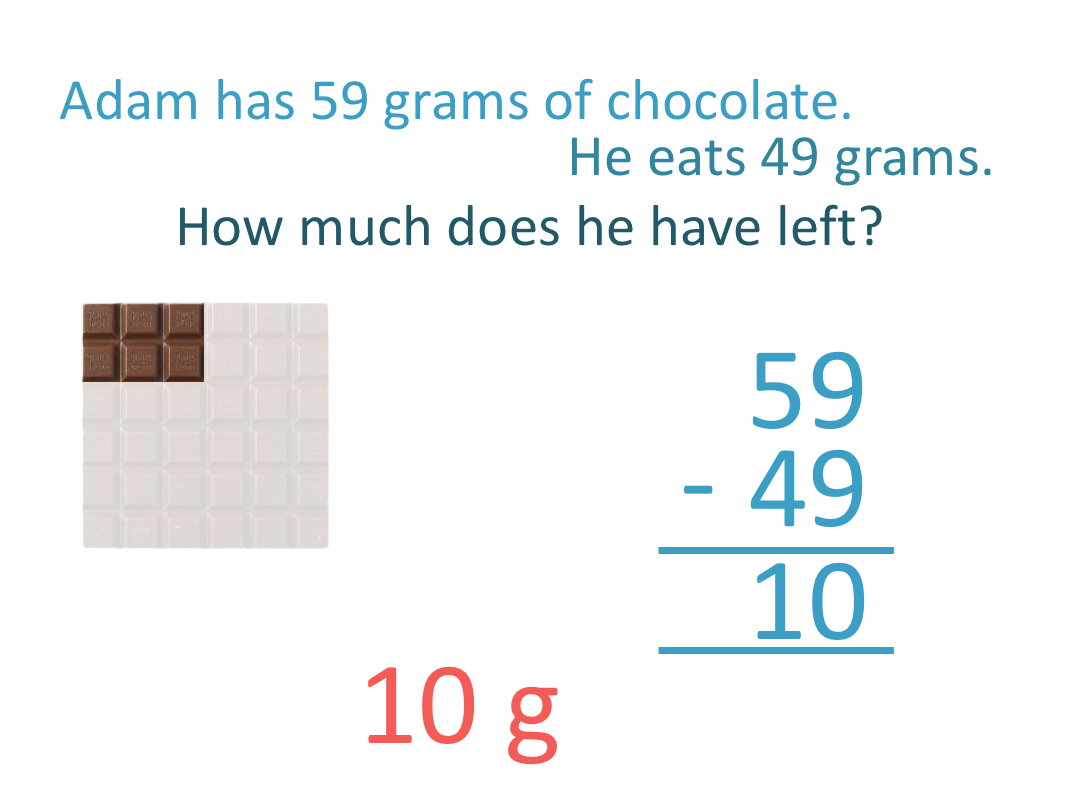There are 10 grams of chocolate remaining.

Here is another word problem example.

I have a candle that is 38 cm long.

After I light it, 11 cm melts away.

How long is the candle now?

This word problem is trickier in that there are not any direct keywords in the question.

However the phrase ‘melts away tells us that we are removing, or subtracting.

Again, when teaching word problems, drawing a diagram is a useful technique.

If no diagram or picture is given, it helps to draw the situation at the start and also the situation at the end.Subtracting the digits in the units column, 8 – 1 = 7.

Subtracting the digits in the tens column, 3 – 1 = 2.

38 – 11 = 27

27 cm of the candle remains.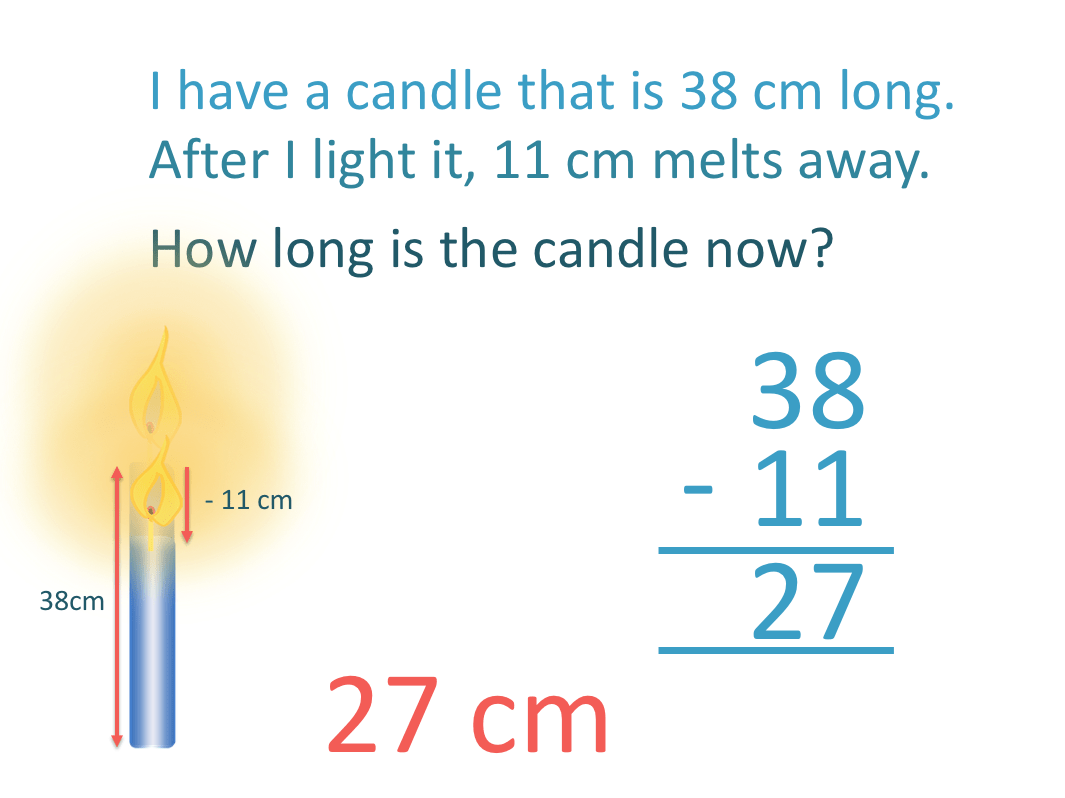In this example, the candle reduced in size because we were removing length as it melted.

The candle has become shorter. Shorter is a word which ends in ‘er’, which can also indicate that we have a subtraction word problem.

‘er’ words often look for a difference between two values and finding a difference is a subtraction.

Again, it can help to draw the situation with a diagram to help understand what type of word problem we have.Now try our lesson on Equivalent Fractions where we learn how to find equivalent fractions by multiplying.